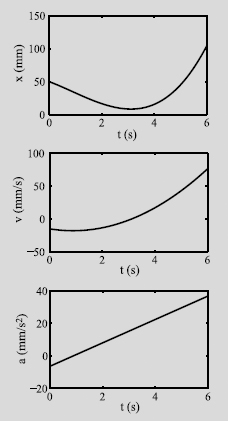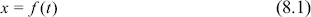# Numerical Differentiation

Core Topics

Finite difference approximation of the derivative (8.2).

Finite difference formulas using Taylor series expansion (8.3).

Summary of finite difference formulas for numerical differentiation (8.4).

Differentiation formulas using Lagrange polynomials (8.5).

Differentiation using curve fitting (8.6).

Use of MATLAB built-in functions for numerical differentiation (8.7).

Complementary Topics

Richardson's extrapolation (8.8).

Error in numerical differentiation (8.9).

Numerical partial differentiation (8.10).Figure 8-1: Position, velocity, and acceleration as a function of time.

## 8.1 BACKGROUND

Differentiation gives a measure of the rate at which a quantity changes. Rates of change of quantities appear in many disciplines, especially science and engineering. One of the more fundamental of these rates is the relationship between position, velocity, and acceleration. If the position, x of an object that is moving along a straight line is known as a function of time, t, (the top curve in Fig. 8-1):the object's velocity, v(t), is the derivative of the position with respect to time (the middle curve in Fig. 8-1):The velocity v is the slope of ...

Get Numerical Methods for Engineers and Scientists 3rd Edition now with the O’Reilly learning platform.

O’Reilly members experience books, live events, courses curated by job role, and more from O’Reilly and nearly 200 top publishers.